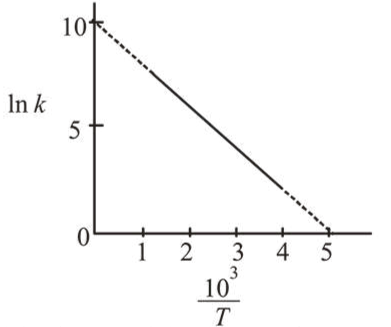# The rate constant (k) of a reaction is measured at different temperatures (T),Question:

The rate constant $(k)$ of a reaction is measured at different temperatures $(T)$, and the data are plotted in the given figure. The activation energy of the reaction in $\mathrm{kJ} \mathrm{mol}^{-1}$ is : ( $R$ is gas constant)1. $2 / R$

2. $1 / R$

3. $R$

4. $2 R$

Correct Option: , 4

Solution:

Arrhenius equation:

$k=A e^{-E a / R T}$\

$\ln k=\ln A-\left(\frac{E_{a}}{R}\right) \frac{1}{T}$

$\ln k=\ln A-\left(\frac{E_{a}}{R \times 10^{3}}\right) \times \frac{10^{3}}{T}$

Slope of graph $=\frac{-E_{a}}{R \times 10^{3}}=\frac{-10}{5}$

$E_{a}=2 R \times 10^{3} \mathrm{~J}=2 R \mathrm{~kJ}$